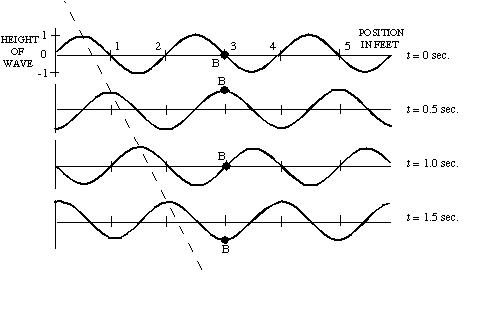TUTORIAL QUESTIONS 60-80Refer to the above image for questions 60-80.

Questions:
60: Medium Velocity
65: Wavelength
70: Amplitude
75: Period
78: Frequency
80: Wave Velocity

60. (See figure above.) The medium velocity is

a) the speed with which the wave moves to the right.
b) the speed with which point B moves up and down.
c) both a and b.

Fundamentals of Sound reference: Secs. 1-D, 1-G.

65.(See figure above.) The wavelength of this wave is
a) 1 ft
b) 2 ft
c) 6 ft
d) cannot be determined

Fundamentals of Sound reference: Secs. 1-D, 1-G.

70. (See figure above.) The amplitude of this wave is
a) 1 ft
b) 2 ft
c) 6 ft
d) cannot be determined

Fundamentals of Sound reference: Secs. 1-D, 1-G.

75. (See figure above.) The period of this wave is
a) .5 second
b) 1 second
c) 1.5 seconds
d) 2 seconds
e) cannot be determined

Fundamentals of Sound reference: Secs. 1-D, 1-G.

78.(See figure above.) The frequency of this wave is
a) 0.5 Hz (or cps)
b) 1 Hz (or cps)
c) 1.5 Hz (or cps)
d) 2 Hz (or cps)
e) cannot be determined

Fundamentals of Sound reference: Secs. 1-D, 1-G.

80. (See figure above.) The propagation velocity (or wave velocity) of this wave is
a) 0.5 ft/s
b) 1 ft/s
c) 1.5 ft/s
d) 2 Hz ft/s
e) cannot be determined

Fundamentals of Sound reference: Secs. 1-D, 1-G.